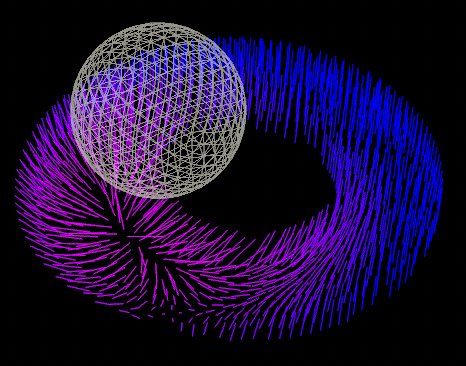## Recommended Posts

Hello,
I'm trying to wrap my head around this... how could I have the orient of nearby points (possibly with a falloff) point avoid (point away from) a nearby object?

My setup right now consists of copied points on a surface and would love them to directional rotate away from the sphere as it gets close to them... here is a scene file if anyone wants to take a stab!

##### Share on other sites

newton's law and try to use this it works

```function float Kernel(vector r ; float h){
float q = length(r);
q /= h;
float factor =  1.0f / (PI  * h * h * h );

if( q>0 && q<=1){
float right =  1.0f - 1.5f  * (q * q) * (1.0f - q/ 2.0f) ;
return factor * right;
}
if(q >1 && q <=2 )
{
float left = factor / 4.0f;
float right = pow(2.0f - q , 3);
return left*right;
}

if(q>2)
{
return 0;
}
return 0;
}

function vector GradKernel(vector r; float h){
float q = length(r);
q /= h;
vector dir = normalize(r);
float factor =  1.0f / (PI  * h * h * h );

if( q<=1){
retgrad = dir * (factor / h) * (-3.0 * q + 2.25f * q*q);
}

if( q<2){
retgrad = dir * (-0.75f * (factor / h) * pow((2.0f - q),2) );
}

}

float h = chf("h");
@P.y = Kernel(@P, h);

Edited by Librarian
•1
•2

##### Share on other sites
1 hour ago, Librarian said:

newton's law and try to use this it works

```
function float Kernel(vector r ; float h){
float q = length(r);
q /= h;
float factor =  1.0f / (PI  * h * h * h );

if( q>0 && q<=1){
float right =  1.0f - 1.5f  * (q * q) * (1.0f - q/ 2.0f) ;
return factor * right;
}
if(q >1 && q <=2 )
{
float left = factor / 4.0f;
float right = pow(2.0f - q , 3);
return left*right;
}

if(q>2)
{
return 0;
}
return 0;
}

function vector GradKernel(vector r; float h){
float q = length(r);
q /= h;
vector dir = normalize(r);
float factor =  1.0f / (PI  * h * h * h );

if( q<=1){
retgrad = dir * (factor / h) * (-3.0 * q + 2.25f * q*q);
}

if( q<2){
retgrad = dir * (-0.75f * (factor / h) * pow((2.0f - q),2) );
}

}

float h = chf("h");
@P.y = Kernel(@P, h);

Thanks! I will take a look appreciate you taking to the time to look.

##### Share on other sites

Hi jon,

this is how you could map distance and direction towards a surface into an orient attribute:

```float dist_min = chf('min_distance');
float dist_max = chf('max_distance');
float angle_max = chf('max_angle');

vector pos = minpos(1, v@P);
float dist = distance(pos, v@P);
vector dir = normalize(pos - v@P);

float mask = 1.0 - smooth(dist_min, dist_max, dist);
vector axis = normalize(cross(dir, v@N));

p@orient = quaternion(angle, axis);```orient_away.hiplc

## Create an account

Register a new account